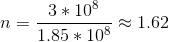# MCAT Physical : Velocity and Index of Refraction

## Example Questions

### Example Question #1 : Velocity And Index Of Refraction

An incandescent light bulb is shown through a glass prism. The certain wavlength of the light is then directed into a glass cuvette containing an unknown concentration of protein. Commonly, this process is called spectroscopy and is used to determine the concentrations of DNA, RNA, and proteins in solutions. The indices of reflection of air, glass, and the solution are 1, 1.5, and 1.3, respectively.

The velocity of the light __________ when it moves from air to glass.

remains the same

decreases

cannot be determined

increases

decreases

Explanation:

This question asks us to consider the relationship between velocity and index of refraction of a medium. If we think back to the definition of index of refraction, we know that it is defined by the ratio of the velocity of light in a vacuum and the velocity of light in some other medium.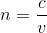n is the index of refraction, c is the speed of light in a vacuum, and v is the speed of light in the new medium.

We can see that n and v are inversely proportional, meaning that the higher the n, the lower the velocity. As the light moved from air (n =1) to glass (n = 1.5), the n increased, and thus the velocity must decrease because the speed of light in a vacuum is constant.

### Example Question #2 : Velocity And Index Of Refraction

An incandescent light bulb is shown through a glass prism. The certain wavlength of the light is then directed into a glass cuvette containing an unknown concentration of protein. Commonly, this process is called spectroscopy and is used to determine the concentrations of DNA, RNA, and proteins in solutions. The indices of reflection of air, glass, and the solution are 1, 1.5, and 1.3, respectively.

As light exits from the wall of the cuvette into the solution, its wavelength __________.

increases

cannot be determined

remains the same

decreases

increases

Explanation:

This question asks us to find the relationship between the wavelength and index of refraction. We will need to know two equations to compute the relationship between the two.

First, we need to relate velocity and index of refraction. The definition of index of refraction allows us to relate the two.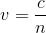We also know the relationship between velocity and wavelength.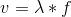We can now set these formulas equal to each other to find the relationship between wavelength and index of refraction.We can see that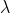and n are inversely related. If n decreases, the wavelength must increase. In our problem, light in moving from a higher index of refraction to a lower one, meaning the wavelength gets longer (increases).

### Example Question #3 : Velocity And Index Of Refraction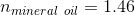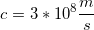How long will it take a photon to travel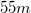through mineral oil?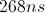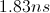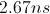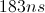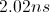Explanation:

The index of refraction is equal to the speed of light in a medium divided by the speed of light in a vacuum.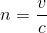We can find the time to travel a given distance by manipulating this equation and combining it with the equation for rate: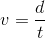.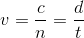Plug in the given values and solve for the velocity in the medium.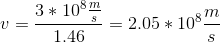Now we can return to the rate equation and solve for the time to travel.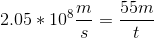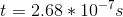We can recognize that the answer can be simplified by converting to nanoseconds.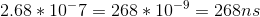### Example Question #4 : Velocity And Index Of Refraction

The refractive index of medium A is 1.2, while that of medium B is 1.36. Through which medium does light travel faster and at what speed does it travel?

The speed of light is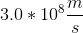.

Medium A at velocity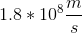The speed of light will bein either medium

Medium B at velocity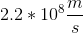Medium A at velocity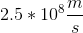Medium B at velocity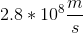Medium A at velocityExplanation:

The refractive index of a medium (n) is equal to the speed of light (c) divided by the velocity of light through the medium (v).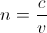Rearranging the equation allows us to see the relationship regarding v.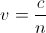The lower the refractive index, the faster the velocity of light. Medium A has the smaller refractive index. Light will travel faster through medium A at a velocity equal to the speed of light divided by the refractive index.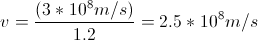### Example Question #5 : Velocity And Index Of Refraction

Which of the following does not take place when a light wave travels from a medium with a high index of refraction into one with a lower index of refraction?

Wavelength increases

Velocity increases

The refracted light remains in phase with the incident wave

Frequency increases

The light ray bends away from the normal line

Frequency increases

Explanation:

As the wave travels into the less dense medium, it speeds up, bending away from the normal line. The index of refraction tells the ratio of the velocity in a vacuum in relation to the velocity the medium; thus, the velocity will be greater in a medium with a lower index of refraction.Frequency remains the same regardless of medium, however, since the velocity changes, the wavelength must accommodate this change.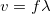If velocity increases and frequency remains constant, wavelength must also increase.

Finally, a phase shift only occurs when a light ray reflects from the surface of a more dense medium.

### Example Question #6 : Velocity And Index Of Refraction

What is the index of refraction for a material in which light travels at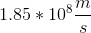?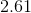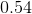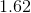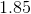Such a material does not exist, since the speed of light is constantExplanation:

Relevant equations: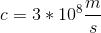To find index of refraction, divide the speed of light in a vacuum by the speed of light in the material: